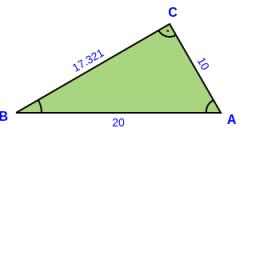# 'Calculate 6224

Right triangle. Given: side c = 15.8 and angle alpha = 73°10'. Calculate side a, b, angle beta, and an area.

a =  15.123
b =  4.5755
B =  16.8333 °
S =  34.5976

### Step-by-step explanation:

Try calculation via our triangle calculator.Did you find an error or inaccuracy? Feel free to write us. Thank you!

Tips for related online calculators